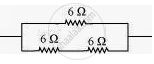# Show how you would connect three resistors, each of resistance 6 Ω, so that the combination has a resistance of 4 Ω. - Science

Numerical

Show how you would connect three resistors, each of resistance 6 Ω, so that the combination has a resistance of 4 Ω.

#### Solution

If we connect the resistors in series, then the equivalent resistance will be the sum of the resistors, i.e., 6 Ω + 6 Ω + 6 Ω = 18 Ω, which is not desired. If we connect the resistors in parallel, then the equivalent resistance will be 6/2 = 3 Ω is also not desired. Hence, we should either connect the two resistors in series or parallel.

Two resistors in series:Two 6 Ω resistors are in series. Their equivalent resistance will be the sum 6 + 6 = 12 Ω.

The third 6 Ω resistor is in parallel with 12 Ω. Hence, equivalent resistance will be

1/(1/12+1/6)=(12xx6)/(12+6)=4Omega.

Therefore, the total resistance is 4 Ω.

Is there an error in this question or solution?
Chapter 12: Electricity - Exercises [Page 221]

#### APPEARS IN

NCERT Class 10 Science
Chapter 12 Electricity
Exercises | Q 11.2 | Page 221
Share